### Home > CALC > Chapter 9 > Lesson 9.3.2 > Problem9-99

9-99.
1. Consider the infinite series below. For each, decide if it converges or diverges and justify your conclusion. If the series converges, find its sum. Homework Help ✎

1. 4 + 4 + 4 + 4 + ...

2.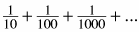3. 10 + 9 + 8 + 7 + ...

4.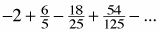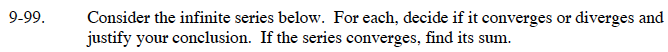For an infinite geometric series: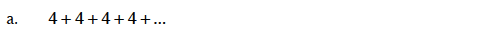This is a geometric series with r = 1.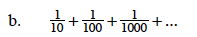This is a geometric series with r = 1/10.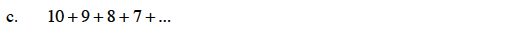This is an arithmetic series. Does an infinite arithmetic series have a finite sum?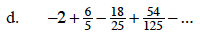This is a geometric series with r = –3/5.